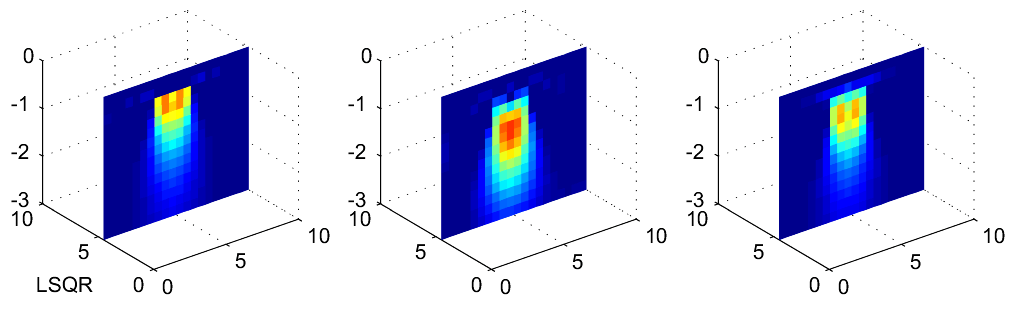Discrete Ill-Posed Problems

# Discrete Ill-Posed Problems

This PhD course at IMM is given by Prof. Per Christian Hansen. MSc students are also welcome; technically, they will follow the course as a DTU "specialkursus".

The aim of the course is to give a practical introduction to the numerical treatment of inverse problems (also known as ill-posed problems) in the form of first-kind Fredholm integral equations, with emphasis on computational and numerical aspects. Therefore, the theory is illustrated by Matlab exercises, in such a way that the student gets hands-on experience with the most common techniques and paradigms. The following topics are treated.

• Inherent difficulties of inverse problems (ill-posed problems).
• Discretization and numerical treatment of integral equations.
• Regularization, stabilization of a solution to an inverse problem.
• Numerical "tools" for the analysis and solution of inverse problems by means of regularization.
• Methods for choosing the regularization parameter (i.e., the amount of regularization).
• Iterative methods for large-scale problems.

### Course Material

• Per Christian Hansen, Discrete Ill-Posed Problems - Insight and Algorithms, A tutorial with Matlab exercises. November 2005.
A preliminary version of these lecture notes is available here.
• The Matlab software package Regularization Tools.